International Journal of Energy and Power Engineering
Volume 5, Issue 1-1, February 2016, Pages: 12-20

Finding Better Solutions to Reduce Computational Effort of Large-Scale Engineering Eddy Current Fields

Dexin Xie1, Zhanxin Zhu2, Dongyang Wu1, Jian Wang2

1School of Electrical Engineering, Shenyang University of Technology, Shenyang, China

2TBEA Shenyang Transformer Co., Ltd., Shenyang, China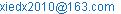(Dexin Xie)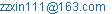(Zhanxin Zhu)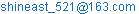(Dongyang Wu)

Dexin Xie, Zhanxin Zhu, Dongyang Wu, Jian Wang. Finding Better Solutions to Reduce Computational Effort of Large-Scale Engineering Eddy Current Fields. International Journal of Energy and Power Engineering. Special Issue: Numerical Analysis, Material Modeling and Validation for Magnetic Losses in Electromagnetic Devices. Vol. 5, No. 1-1, 2016, pp. 12-20. doi: 10.11648/j.ijepe.s.2016050101.12

Abstract: In the finite element analysis of the engineering eddy current fields in electrical machines and transformers there are the problems such as the huge scale of computation, too long computing time and poor precision which could not meet the demand of engineering accuracy. The current research situation and difficulties of these problems are analyzed in this paper mainly from the aspect of computation methodology. The methods to deal with these problems, e.g., homogenization models of the laminated iron core, the sub-domain perturbation finite element method, domain decomposition method, and EBE (Element by Element) parallel finite element method are described. Their advantages and limitations are discussed, and the authors’ suggestions for the further research strategies are also included.

Keywords: Engineering Eddy Current Fields, Huge Scale of Computation, Homogenization of Laminated Iron Core, Finite Element Method, Sub-Problem Perturbation Finite Element Method, EBE Parallel Finite Element Computation

1. Introduction

The electromagnetic fields in most of the electrical devices, such as electrical machines and transformers, are classified as quasi-static field. The quasi-static electromagnetic field in which conductive materials are included is called eddy current field too. It is of great significance to calculate the distribution of the eddy current field with its losses induced in the conductive materials accurately for optimal design and safe operation of the electrical devices. As an example, there are many metal structural components in power transformer. The eddy current losses in the components induced by the variation of leakage magnetic field are one of the heating sources for temperature rise. Although decreasing the whole losses in the structural components is important, the local loss concentration due to non-uniform distribution of the losses is the direct reason of local over-heating and operating faults, which deserve to pay close attention. Even if the computational technology has got rapid development in nowadays, to improve the computation precision is still a difficult task for numerical analysis of eddy current fields in super-huge type of power transformers. The features of this kind of computation include very small skin depth of ferromagnetic material, nonlinear and anisotropic electromagnetic characteristics of the structure components, structural discontinuity of the laminations, three dimensional feature of the spacial configuration, non-sinusoidal variation of the field physical quantities, and so on. Therefore, although there have been some commercial softwares of electromagnetic field analysis used in manufacture enterprises of power transformers extensively for performance verification and aided design, the calculated results are not agree well with the experimental ones. The crux of the problem is in the aspects of the conflict between the huge computational scale and high precision required, the difficulty of magnetic characteristic modeling for laminated iron core, the higher harmonics of exciting electric current and magnetic field being not easy to included, and lack of the data which can describe the electromagnetic characteristics of materials of the structural components accurately and completely. The aim of this paper is to analyze the current study situation of these problems, discuss the research strategies, and propose the further research directions from the authors’ point of view.

2. Simulation of the Material Characteristics of Laminated Iron Core

2.1. Difficulty of the Magnetic Characteristic Modeling of Laminated Iron Core

Proper modeling of material characteristics is the basis to improve the accuracy of electromagnetic field computation. However, it is not easy to simulate the electromagnetic characteristics of laminated iron core. The iron core and magnetic shield of power transformer consist of grain-oriented silicon steel sheets laminated, and the thickness d of each sheet is around 0.3 mm or even less, see Fig. 1(a). In the plane of the sheet and the angles with the rolling direction ranging from 00to 900, the magnetic characteristics including permeability and loss per kg are angle-dependent anisotropic, which need to be depicted by the so-called two dimensional magnetic characteristic model. M. Enokizono and N. Soda proposed the well-known E&S model  in 2000 and kept improving it. With this model not only the loss in silicon steel sheets resulted from alternating magnetic field can be calculated, but the losses due to local rotating magnetic field can also be computed. In order to incorporate the E&S model into finite element (FE) analysis the 2D magnetic characteristic test device  has to be used to obtain a great deal of data for different angles in the sheets, different exciting levels and moments of a sinusoidal period, as the computation basis. However, this model has been confined to 2D computation [3, 4], not being extended to 3D yet. The reason is that at first the specialized 2D test device has not been used commonly, and more importantly this model involves time-discretization and iterations, which will cause the calculational scale over-increasing thus lead to unworkable computation effort if the model is used in 3D analysis directly. Furthermore, for 3D analysis more factors have to be considered, e. g., the material nonuniformity of the laminated iron core, the increasing of the unknowns, the ill-conditioned degree of the coefficient matrix, and so on.

It is known that for the magnetic field in iron core under a sinusoidal excitation when the direction of the magnetic field is parallel to the surface of the sheet the distribution of its magnitude and phase are nonuiform along the thickness of the sheet. At this condition the 1D classical analytic solutions of flux density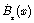and eddy current density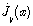are given as [5, 6]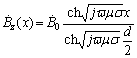(1)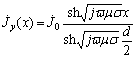(2)

where x stands for the coordinate along the direction perpendicular to the sheet,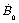and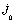are the amplitude ofandat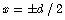respectively.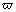,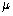,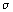are angle frequency, permeability and conductivity respectively. Fig. 1(b) shows the magnitude of flux density and eddy current density versus x respectively, in which the component of magnetic field perpendicular the sheet is neglected, although it exists in practical operating condition.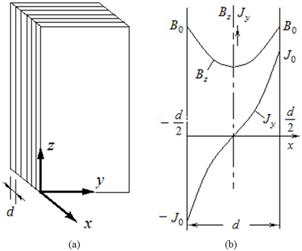Figure 1. (a) Sketch map of laminated iron core (b) Bz and Jy versus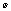.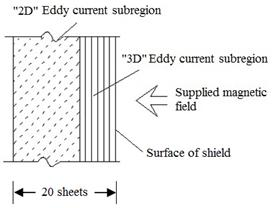Figure 2. FEM model of the eddy current in laminated sheets of magnetic shield .

Considering the nonuniformity of the magnetic field in the thickness of the sheet, the sheet itself should be meshed in 3D FE analysis. It has been done so as in , in which the TEAM Problem P21-M1/M2 is chosen as a numerical model and the single sheet of magnetic shield is meshed. The magnetic shield is made of 20 sheets with a thickness of 0.3mm for each in the model, and the supplied magnetic field is perpendicular to the surface of the sheets, as shown in Fig. 2. According to the results of experiment, the distribution of magnetic field in the 6 sheets which are near the surface of the shield is three dimensional, while in the other sheets further from the surface the magnetic field direction is parallel to the sheet surface basically. Therefore, the laminated region is divided into two sub-regions of "2D" and "3D" in 3D FE analysis, see Fig. 2. In the "3D" sub-region each sheet is meshed into 3 layers, and the insulation layer between two sheets is also meshed with one layer, while for the "2D" sub-region the electromagnetic characteristics are given using more rough meshes with the conventional homogenization of iron core material, which has been used extensively and will be illustrated in Section 2.2. The calculated results of this model satisfied the demand of engineering precision basically. However, the model is too small compare with the practical huge transformer products. For the latter, the so refined meshes lead to over huge computational scale so that the computation task cannot be fulfilled.

2.2. Conventional Rough Homogenization of Laminated Iron Core

To avoid too large scale of computation in FE analysis the simplified modeling method for the electromagnetic characteristics of the laminated iron core has been used commonly, that is, taking the laminated stack as a continuum and its electromagnetic characteristics are given uniformly as follows, i. e.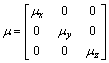(3)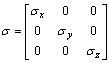(4)

whereandare permeability and conductivity respectively, and their components can be determined, depending on the connection in series or in parallel of the silicon steel sheets and insulation layers according to the magnetic and electric circuit theory. After proper simplifying the values of the components can be given as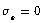,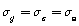,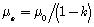,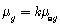,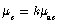for the laminated direction shown in Fig.1(a), where k is the lamination coefficient,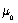is the permeability of vacuum, and the subscript s stands for silicon steel sheet. This method has been used extensively, and some commercial softwares of electromagnetic field analysis also use the method in a similar way. However, for the computation of eddy current field and its losses due to leakage magnetic field in practical transformer products this modeling of the homogenization is too rough to obtain satisfied results.

2.3. New Development of Magnetic Characteristic Modeling of Laminated Iron Core

Reference  proposes a more refined homogenized method for laminated iron core, with which the eddy current density in silicon steel sheet is divided into two components, that is, the component 1 induced by the alternating flux density parallel to the sheet and the component 2 due to the flux density perpendicular to the sheet. To incorporate the method into 3D FE analysis of magnetic vector potential and electric scalar potential scheme, for the component 2, the electromagnetic characteristic modeling is the same as that described in Section 2.2, the conventional homogenization, while for the component 1, an addition item is added into the Galerkin weak formulation.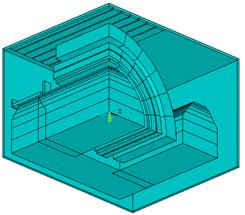(a)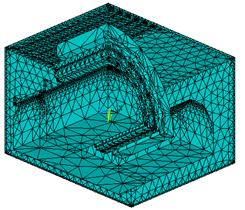(b)

Figure 3. Single phase transformer model of 380MVA/500 KV (a) Structural diagram (b) Meshes of the transformer model.

For the low frequency case, the addition item is given as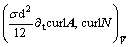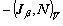(5)

where N is the basis function, A is the magnetic vector potential,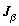corresponds to the eddy current density generated by the flux density parallel to the sheet, which is regarded as a kind of source electric current density, and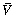is the laminated region. The deducing of (5) is on the condition that the skin effect in a sheet is neglected, i. e. the distribution of flux density along the thickness of a sheet is uniform which equals to the average flux density, and the distribution ofis linear. That is, contrasting with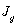in Fig. 1(b),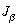is a straight line instead of a curve in this case. The method is used in a numerical model of laminated stack with FE computation in frequency domain and a satisfied result is obtained . For more complicated calculated model, a practical product model of a 380MVA/500 KV single phase power transformer with the engineering frequency of 50 Hz is used by the authors, and its structure and FE meshes are shown in Fig. 3. The method proposed in  is extended to time domain and nonlinear permeability is considered [10, 11], the calculated total losses due to leakage magnetic field are agree with the experimental values and the error is around 6%. Figure 4 shows parts of the numerical results, i. e. the flux density distribution on the symmetry plane and the eddy current density distribution on the oil tank inner surface of the transformer model. It is obvious that with this method the good computed results are obtained with relative less and acceptable computational effort.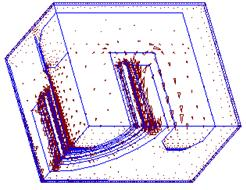(a)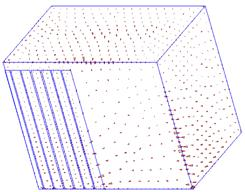(b)

Figure 4. (a) Flux density distribution of the symmetry plane of the transformer, (b) Eddy current density distribution of the oil tank inner surface of the transformer.

For higher frequency, the skin effect cannot be neglected. A more accurate homogenization method is presented by . Based on the classical analytic solutions of magnetic flux density and eddy current density, i.e. (1) and (2), a more complicated addition item is put into the weak formulation to simulate the eddy current generated by the flux density parallel to the sheet. Furthermore, the method has been extended from frequency domain to time domain, and the insulating layers of finite width between the laminated sheets are considered too . The test numerical model is a linear 3D axisymmetrical one and a good result is achieved. A quantitative conclusion is drawn by  that the simplified model for the low frequency case is valid up to a frequency for which the skin depth is equal to the half-thickness of the laminations, while the accurate model is valid for any frequency. However, till now the method hasn’t been used in practical engineering model yet.

3. Transformation of Global Solution to Combination of Partial Solutions

Since the computational scale is very large for a global solution, trying to substitute combination of a certain partial solutions with less computational effort for global solution may be a novel strategy, among which the sub-problem perturbation FE method and domain decomposition method are both typical examples.

3.1. Sub-Problem Perturbation FE Method

The so-called perturbation FE method is proposed initially in . The method is applied to eddy current nondestructive evaluation problems. To probe the flaw of metallic tube using external magnetic field created by exciting coil a 3D eddy current field calculation has to be carried out. However, compared with the dimension of the coil and tube, the size of the flow is too small, which results in the conflict of computation scale and accuracy. In fact, the computation of the tube eddy current field is much easier for the case without the flaw, e. g., through analytical solution, or adopting FE calculation of axisymmetric filed based on the symmetry of the tube, but when computing the eddy current field with the flaw, the known solution without the flaw cannot be used. To solve the problem an ingenious method is presented by . The main idea is that the practical eddy current field with the flaw involves three fields, denoted here as field 0 for the tube with the flaw, field 1 for that without the flaw, and field 2 the perturbed field created by the flaw. The governing equations of field 2 can be obtained by subtracting the governing equations of field 1 from that of field 0. The 3 governing equation systems are all Maxwell equations, however, there is an additional item in the right side of the equations of field 2. Taking the curl equation of magnetic field as an example, that is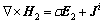(6)

Equation (6) is the perturbation equation of magnetic field, where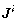=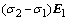, called as incident electric current density. It iswhich causes the perturbation to field 1, and the perturbation results from the conductivity change of the flow region. H and E are magnetic field intensity and electric field intensity respectively, subscript 1 and 2 correspond to field 1 and 2 respectively,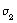is the conductivity of the flaw region, and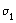is that of the region outside of the flaw. Obviously the field of the tube with flaw, the field 0, is equal to the sum of the field 1 and field 2 for the case of linear electromagnetic characteristics. The advantage of this perturbation FE method is that the solution of field 1 can be obtained easily; the computation of field 2 can be carried out using conventional FE method and is independent of field 1. Furthermore, the computational domain and mesh size of the field 2 are much less than that of field 1, because the affected area of the flaw is not very large, in general beyond 5~6 skin depth the flaw field may considered zero.

Getting the hint of perturbation FE method in nondestructive testing, reference  extends the method to the computation of the skin and proximity effects in conductors of any properties and shapes, in both frequency and time domains. Because two sub-problems have to be calculated, the developed method could be called as sub-problem perturbation FE method. The main points are as follows. The practical eddy current field (field 0) is regarded as the superposition of reference field (field 1) and perturbation field (field 2). The field 1 and field 2 are calculated respectively, and their computational effort is much less than that of computing the field 0 directly. The calculation methods in two cases in which the conductive or magnetic material is included in the solved domain, are proposed in , which will be described in Section 3.1.1 and 3.1.2 respectively and briefly.

3.1.1. Case of Electric-Conductive (Non-Magnetic) Material Included

Reference field (field 1): Set the solid conductive material as ideal conductor, i.e., the conductivity of which equals to infinite, so that the conductor can be excluded from the solved region. Provide that the normal component of magnetic flux density is equal to zero at the boundary of the region.

Perturbation field (field 2): Include the conductive domain in the solved region, in which the practical conductivity is assigned. Consider the difference of the conductivity from that of ideal conductor as the perturbation to field 1. At the interface of conductive and non-conductive domains set the tangential component of magnetic intensity as that of the calculated results of field 1, which is taken as the source of the perturbation field.

3.1.2. Case of Magnetic (Non-Electric-Conductive) Material Included

Reference field (field 1): Set the solid magnetic material as ideal magnetic conductor, i.e., the permeability of which equals to infinite, so that which is removed from the solved region. At the boundary adjacent to the ideal magnetic conductor the tangential component of magnetic intensity is set as zero.

Perturbation field (field 2): Include the magnetic material in the solved region, in which the practical permeability is assigned. Assign the normal component of flux density as that of the calculated results of field 1 at the interface of the magnetic and non-magnetic domains, which is taken as the source of the perturbation field.

3.1.3. Discussion

In the two cases described in Section 3.1.1 and Section 3.1.2 the solutions of the practical problem are all equal to the superposition of the solutions field 1 and field 2. For the calculation of field 1 the computational scale is reduced because that the conductive or magnetic region is removed so that the pressure of mesh generation is decreased. For the calculation of field 2 the solved region can be reduced as explained in Section 3.1. Fig. 5 and Fig. 6 are two numerical examples given by  for the two cases respectively.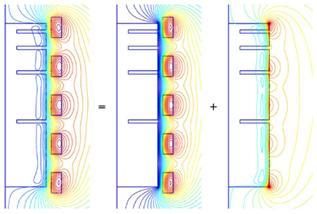Figure. 5. Magnetic flux lines of the system with a conductive non-magnetic core . Left: for the conventional FE solution, Middle: the reference solution, Right: the perturbation solution.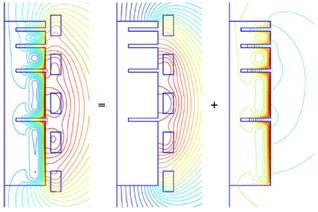(a)                   (b)                 (c)

Figure 6. Magnetic flux lines of the system with a magnetic conductive core (non-electric conductive) . (a) for the conventional FE solution, (b) the reference solution, (c) the perturbation solution

Compared with the well-known Surface Impedance Method [15-17], Sub-problem Perturbation FE Method can describe the field inside the conductors in more detail. However, it worth noting that there is still room for studying the method further, e. g., in fact the silicon steel is not only magnetic but also electric conductive material, therefore when considering both the magnetic and electric parameters at the same time, how to deal with the problem? Besides, the principle of superposition is valid only in the linear cases, then how to incorporate the nonlinearity of the material parameters in the calculation? Even though there are the questions to be answer, the method offers a very good idea to solve complicated problems.

3.2. Domain Decomposition Method

Domain decomposition method  is the one with which the solved domain of a definite-solution problem of partial differential equation is divided into two or more than two subdomains. The method itself can be classified overlapping domain decomposition method and non- overlapping domain decomposition method according to that the subdomains are overlapping or not. The solution of each subdomain is conducted independently, and the discretized meshes of subdomains are independent of each other. The interaction of the subdomains is dealt with by means of the iterations of certain interface conditions. In this way, the solution of the original problem for global domain is transformed into the solutions of subdomains, so that the computational scale is reduced greatly.

3.2.1. Overlapping Domain Decomposition Method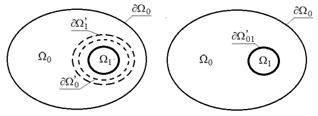(a)                                (b)

Figure 7. Sketch map of domain decomposition (a) Overlapping domain decomposition method; (b) Non-overlapping domain decomposition method.

Overlapping domain decomposition method (ODDM) is based on the so-called Schwarz alternating method. There are various choices for the domain division strategy due to the difference of the concrete problems. One of the division modes is shown in Fig. 7(a), where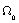is the global domain of the original problem, and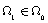is the interested domain. In the calculating process of the domain decomposition, denote the subdomain between boundary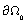and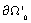as subdomain 1 while the subdomain surrounded by boundary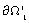as subdomain 2, and the calculation of the two subdomains are conducted separately. The numerical boundary conditions are given alternately at the boundaryandtill the iterative solutions at these boundaries satisfy the convergent condition. A typical calculating procedure is given as follows.

1. Carry out the FE calculation in global domainwith coarse meshes then retrieve the discrete solution at.

2. Calculate the FE solution in subdomain 2 with refined meshes. The Dirichlet condition at the outside boundaryof the subdomain 2 is provided by step 1 at the first iteration and by step 3 at and after the second iteration.

3. Calculate the FE solution in subdomain 1 with coarse meshes. The boundary condition at the inside boundaryis provided by step 2.

4. Calculate the iteration error of the solution atandrespectively. If the error criterion is met then stop, or go to step 2.

It should be pointed that the setting of step 1 is to accelerate the convergence, which is not a necessary step. If the whole computation begins with the step 2, the boundary condition atcould be set arbitrarily.

3.2.2. Non-Overlapping Domain Decomposition Method

The sketch map of non-overlapping domain decomposition method (NODDM) is shown in Fig. 7(b). It is obvious from the figure that there is no overlap between the two subdomains,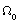and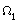. Their interface,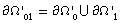, can be regarded as the limit case of the overlapping area reduced in Fig. 7(a). The calculation steps of the NODDM are similar as that of the ODDM. However, in the calculation of the former the Dirichlet and Neumann boundary conditions are alternately adopted in general. For a same problem, the convergence speed of the ODDM is faster than that of the NODDM. Furthermore, the lager the overlapping area, the faster the convergence, but the greater the calculation scale of each subdomain. Therefore, it is necessary to balance the convergence speed and the calculation scale of the subdomains properly and choose the division of overlapping or non-overlapping subdomains reasonably.

3.2.3. An Example to Compare the Computation Time

To compare the computation time of the domain decomposition method with conventional FE method, a small model, the P21a -0 of TEAM-based Benchmark Family  is calculated using the ODDM . Table 1 lists the discretization data of the model for two subdomains, and Table 2 shows the comparison of calculated results of the model with the ODDM and conventional FE Method. It can be seen from Table 2 that at similar accuracy level the computation time with ODDM reduced to a quarter of that with the conventional method.

3.2.4. Discussion

The domain decomposition method has been applied in fluid dynamics problems, wave and Laplace equation problems more commonly [19-21], but not been used to eddy current field computation very often. For the FE analysis of nonlinear and anisotropic eddy current field the use of the method is restricted to the model with relatively simple and regular structure . It should be noted that when using the method to analyze 3D eddy current field of large scale transformer products the boundary positions of subdomains have to be chosen carefully to avoid the abrupt change of electric and magnetic parameters in the boundary that could result in poor convergence performance. Therefore, the division of subdomains for practical products with complicated structure becomes troublesome, which is an obstacle to the application of the domain decomposition method in this field.

Table 1. Discretization Data of P21a-0 Model for Two Subdomains.

 Name of Subdomain Subdomain 2 (Interested) Subdomain 1 Type of element Hexahedron of 8 nodes Hexahedron of 8 nodes Number of elements 18816 20844 Number of nodes 21315 18161 Number of unknowns 71820 54204 Non-Zero Elements of Matrix 3066446 2019909

Table 2. Comparison of Calculated Results of P21a-0 Model with the ODDM and Conventional FE Method.

 Measured Losses (W) Calculated Losses (W) Error (%) Computation Time (h) Conventional 9.17 9.233 0.687 2.0 ODDM 9.17 9.245 0.818 0.5

4. New Development of Parallel Algorithm-EBE Method

Parallel algorithm is a powerful tool to deal with the large scale computation in engineering domain. The parallel methods for FE analysis include mainly the parallel solution of FE equations, the parallel of domain decomposition, and the parallel based on element level, i.e., Element by Element (EBE) parallel FE method.

The parallel solution of FE algebraic equations has been adopted in different areas, but its parallel efficiency is not very high. Especially for the large scale computation of an engineering problem the requirement of memory rises steeply, then when conducting the conventional CPU-based calculation the date interchange have to be performed, which makes the computation time for that increased greatly, so that the calculating speed declines [22-23]. The limitations of the domain decomposition method have been stated in Section 2.2.

The EBE FE method based on the element-level is an effective method to improve the degree of parallelism at the algorithm-level. With this method the main calculation can be performed independently and parallelly for each element, and only in limited stages the correlation and transmission of the element data should be carried out. Therefore, it doesn’t need to create and store the global coefficient matrix, thus the requirement of memory is reduced greatly. The more great the computational scale, the more obvious the effect by using the method, so that this method is especially suitable for the numerical computation of engineering electromagnetic field problems with complicate structure and huge computational scale. The method is proposed initially by  in1983, and is applied to the domains of heat conduction, solid mechanics and structural mechanics gradually [25-26].

At present the research of EBE method has come to a stage of combining algorithm, software and hardware. In recent years, based on the Graphic Processing Units (GPU), the General Purpose Computation on GPUs (GPGPU) has developed rapidly. Furthermore, the arising of the Compute Unified Device Architecture (CUDA)  supplies a reliable programming environment for the realization of the GPGPU, which provides a certain condition for the parallel computation of large-scale engineering problems. Currently, the GPU has been applied to electrical system, graphic processing, mechanics [28-32], etc..

For the application of the EBE algorithm to electromagnetic field it is still confined to the problems of static field  now. Because of the specificity of eddy current problems, the EBE parallel FE Method with the GPU computing platform is not applied in the solution of the eddy current fields yet so far. The key point is that the implementation of EBE method is combined with Conjugate Gradient (CG) method, but the convergence performance is poor when using the CG method to solve the FE equations of eddy current field. The main calculation of the CG method involves the product of matrix and vector, which is particularly suitable for parallel computation, but the use of the method should be based on the condition that the coefficient matrix of the solved equations is positive definite and symmetric. For the boundary value problem of Laplacian equation, the EBE method can be used successfully because that the condition is satisfied. As an example, it is shown that for a 2D coaxial-cable model of static electric field using the EBE parallel calculation with the multi-core GPU of the first and third generations the computational speed is accelerated 14 and 111 times respectively than that of the serial calculation with CPU. However, for the eddy current problem, although the coefficient matrix of FE discretized equations is symmetric, which is not positive definite, so that the precondition of applying CG method is dissatisfied. Furthermore, when solving 3D eddy current field by using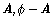or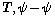scheme which is adopted commonly at present, the coefficient matrix of the resultant FE equations is ill-conditioned. In this case if the CG method is still used to solve the equations, the convergence performance will get worse greatly, or even a stable solution cannot be obtained. This is the major obstacle to use EBE method for solution of eddy current field. To overcome the obstacle it is necessary to start with mathematical model, preprocessing of parallel CG method, etc. The attempt of this aspect is now in progress .

5 Conclusions

This paper starts from the current problems in large scale eddy current field FE computation of electrical machines and transformers, then analyzes the present research situation in this domain, expounds the main difficulties to solve the problems, introduces several methods for reducing computational effort and improving calculating accuracy, e.g., the material performance homogenization model of laminated iron core, sub-problem perturbation FE method, domain decomposition method, and EBE parallel FE method, at the same time indicates the advantages and limitations of the different methods. In the methods above mentioned the EBE parallel FE method is a powerful tool to implement the accurate computation of 3D eddy current field by the authors opinion, but in order to realize the computation it is still necessary to solve a set of problems. Therefore, more efforts still need to be put into exploring further the ways to fulfill the computation task rapidly and effectively by drawing lessens from and synthesizing the research results with the above methods.

References

1. N. Soda, and M. Enokizono. "Improvement of T-Joint part constructions in three-phase transformer cores by using direct loss analysis with E&S model". IEEE Trans. Magn., Vol. 36, No. 4, pp. 1285-1288, 2000.
2. E. Enokizono, T. Suzuki, J. Sievert, and J. Xu. "Rotating power loss of silicon steel sheet". IEEE Trans. Magn., Vol. 26, No. 5, pp. 2562-2564, 1990.
3. YanliZhang, Houjian He, Dexin Xie, and Chang-seop Koh."Study onvectormagnetic hysteresis model of electrical steel sheets based on two-dimensional magnetic property measurement,"Proceedings of the CSEE, Vol. 30, No. 3, pp. 130-135, 2010. (in Chinese)
4. YanliZhang, Houjian He, Dexin Xie, and Chang-seop Koh."Finite element analysis of magnetic field in transformer core coupled with improved vector hysteresis model and its experimental verification,"Proceedings of the CSEE, Vo. 30, No.21, pp. 109-113, 2010. (in Chinese)
5. K. Simonyi. Theoretische Electrotechnik, VEB Deutscher, Verlag, 1956.
6. Yunqiu Tang. Electromagnetic Field in Electrical Machines. (Second edition),Beijing:Science Press,1998. (in Chinese)
7. Zhiguang Cheng,Norio Takahashi,Behzad Forghani et al. Electromagnetic and Thermal Field Modeling and Application in Electrical Engineering. Beijing, Science Press,2009. (in Chinese)
8. Dexin Xie et al. Finite Element Analysis of Three Dimensional Eddy Current Field. Second edition. Beijing:China Machine Press,2007. (in Chinese)
9. Patrick D,andJohan G. "A 3-d magnetic vector potential formulation taking eddy currents in lamination stacks into account,"IEEE Transactions Magn.,Vol. 39, No. 3, pp. 1424-1427,2003.
10. Zhanxin Zhu, Dexin Xie, and Yanli Zhang. "Time domain analysis of 3D leakage magnetic field and structural parts loss of large power transformer,"Proceedings of the CSEE,Vol. 32, No. 9, pp. 156-160, 2012. (in Chinese)
11. Zhanxin Zhu. Research on Calculation Method of 3D Eddy Current Field Structural Part Losses in Large Power Transformer, Doctoral Dissertation, Shenyang:Shenyang University of Technology,2012. (in Chinese)
12. J. Gyselinck and P. Dular. "A Time-domain homogenization technique for laminated iron cores in 3-D finite-element models," IEEE Trans. Magn., Vol. 40, No. 2, pp. 856-859, 2004.
13. Zsolt Badics, Yoshihiro Matsumoto, Kazuhiko Aoki, Fumio Nakayasu, Mitsuru Uesaka, and Kenzo Miya. "An affective 3-D finite element scheme for computing electromagnetic field distortions due to defects in eddy-current nondestructive evaluation," IEEE Trans. Magn., Vol. 33, No. 2, pp. 1012-1020, 1997.
14. Patrick Dular, Ruth V. Sabariego, and Laurent Krähenbühl. "Subdomain perturbation finite-element method for skin and proximity effects," IEEE Trans. Magn., Vol. 44, No. 6, pp.738-741, 2008.
15. S. A. Schelkunoff, "The impedance concept and its application to problems of reflection, shielding and power absorption," Bell System Technical Journal, pp. 17-48,1938.
16. E.M. Deeley, Serface "Impedance near edges and corners in three-dimensional media," IEEE Trans. Magn.,Vol. 26, No. 2, pp. 712-714, 1990.
17. Yong-Gyu Park et al. "Three dimensional eddy current computation using the surface impedance method considering geometric singularity," IEEE Trans. Magn.,Vol. 31, No. 3, pp. 1400-1403, 1995.
18. Tao Lv, Jimin Shi, and Zhenbao Lin. Domain Decomposition Algorithms—New Technology of Numerical Solution of Partial Differential Equation.Beijing:Science Press, 1997. (in Chinese)
19. R. Glowinski, Q. V. Dinh, J. Periaux. "Domain decomposition methods for nonlinear problems in fluid dynamics," Computer Methods in Applied Mechanics and Engineering, Vol. 40, No. 1, pp. 27-109, 1983.
20. Zhu Z. H., Ji H., and Hong W."An efficient algorithm for the parameter extraction of 3D interconnect structures in the VLSI circuits domain decomposition method," IEEE Transactions on Microwave Theory and Techniques, Vol. 45, No. 9, pp. 1179-1184, 1997.
21. Hanqing Zhu. Study on the Applications of Domain Decomposition Method in Electromagnetic Problems,Doctoral Dissertation, Chengdu:University of Electronic Science and Technology of China,2002. (in Chinese)
22. Mifune T, Iwashita T,and Shimasaki M. "A fast solver for FEM analysis using the parallelized algebraic multi-grid method,"IEEE Trans. Magn.,Vol. 38, No. 2, pp. 369-372, 2002.
23. Steve McFee, Qingying Wu, Mark Dorica, et al. "Parallel and distributed processing for h-p adaptive finite-element analysis: a comparison of simulated and empirical studies,"IEEE Trans. Magn., Vol. 20, No. 2, pp. 928-933, 2004.
24. Hughus T J R, Levit I, and Winget J. "An element-by-element solution algorithm for problems of structural and solid mechanics," Computer Methods in Applied Mechanics and Engineering,Vol. 36, pp.241-254, 1983.
25. Shunxu Wang, Boguo Sun, and Shuquan Zhou. "A mixed EBE parallel algorithm for transient heat conduction problems,"Journal of Huaihai Institute of Technology,Vol. 8, No. 3, pp. 7-9,1999.
26. Yaoru Liu, Weiyuan Zhou, and Qiang Yang. "A distributed memory parallel element by element scheme based on Jacobi-conditioned conjugate gradient for 3D finite element analysis,"Finite Elements in Analysis And Design, Vol. 43, pp. 494-503, 2007.
27. Shu Zhang, Yanli Chu. CUDA of GPU High Performance Computation, Beijing:China Water Power press,2009. (in Chinese)
28. Han Jiangand Quanyuan Jiang. "A two-level parallel transient stability algorithm for AC/DC power system based on GPU platform,"Power System Protection and Control, Vol. 40, No.21, pp. 102-108, 2012. (in Chinese)
29. Youquan Liu, Kangxue Yin, and Enhua Yin. "Fast GMRES-GPU solver for large scale sparse linear systems. Journal of Computer-Aided Design & Computer Graphics,"Vol. 23, No. 4, pp. 553-560, 2011. (in Chinese)
30. Xiaohu Liu, Yaoguo Hu, and Wei Fu. "Solving large finite element system by GPU computation," Chinese Journal of Computational Mechanics,Vol. 29, No. 1, pp.146-152, 2012. (in Chinese)
31. Barnat, J., and Bauch, P. "Employing multiple CUDA devices to accelerate LTL model checking," IEEE 16th International Conference on Parallel and Distributed System, pp. 259-266, 2010.
32. Thurley, M.J. and Danell, V. "Fast Morphological Image Processing Open-Source Extensions for GPU Processing with CUDA," IEEE Journal of Selected Topics in Signal Processing, Vol.6, No. 7, pp. 849-855, 2012.
33. David M.Fernández, Maryam Mehri Dehnavi, and Warren J.Gross. "Alternate Parallel Processing Approach for FEM," IEEE Trans. Magn., Vol.48, NO.2, pp. 299-402, 2012.
34. Renyuan Tang, Dongyang Wu, and Dexin Xie."Research on the key problem of element by element parallel FEM applied to engineering eddy current analysis,"Transaction ofChina Electrotechnical Society, Vol.29, No.5, pp. 1-9, 2014.

 Contents 1. 2. 2.1. 2.2. 2.3. 3. 3.1. 3.2. 4. 5
Article ToolsAbstractPDF(1543K)